# Precalculus : Graph Equations

## Example Questions

### Example Question #1 : Cartesian Coordinate System

Given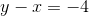, which graph is the correct one?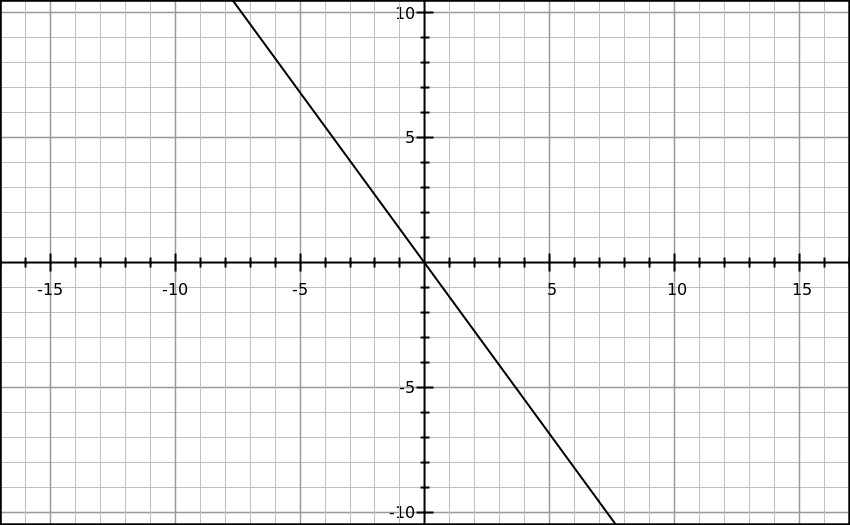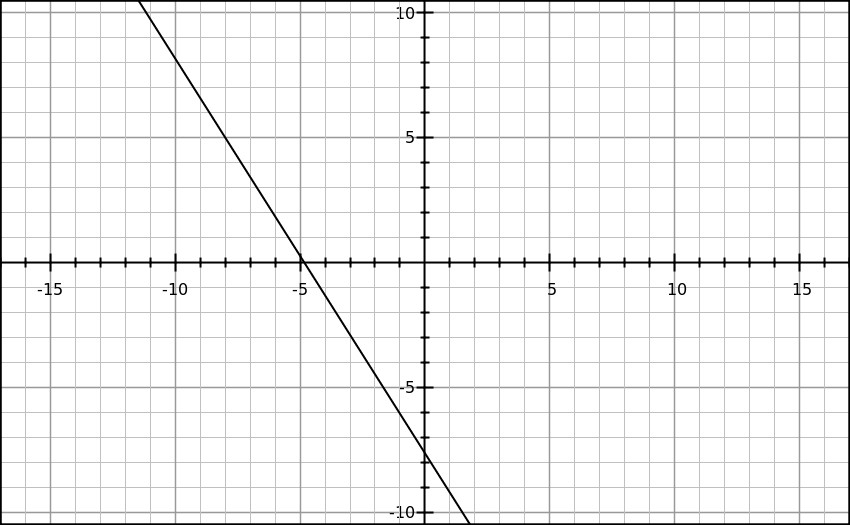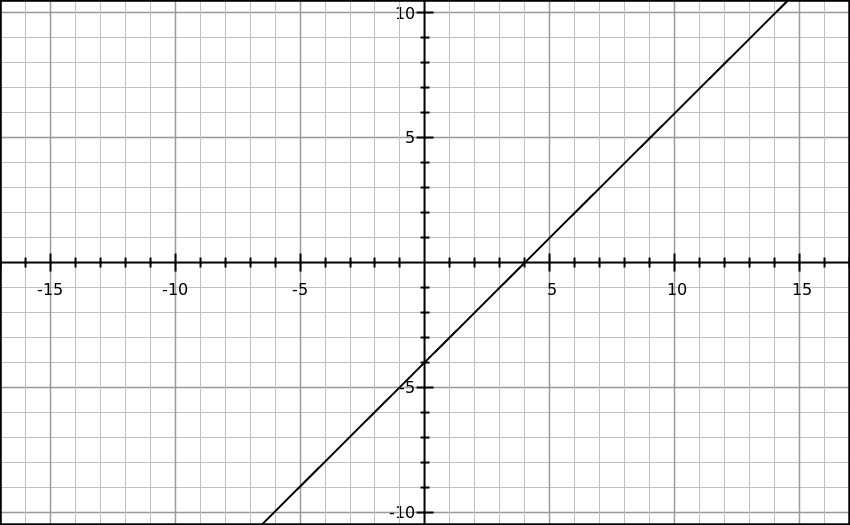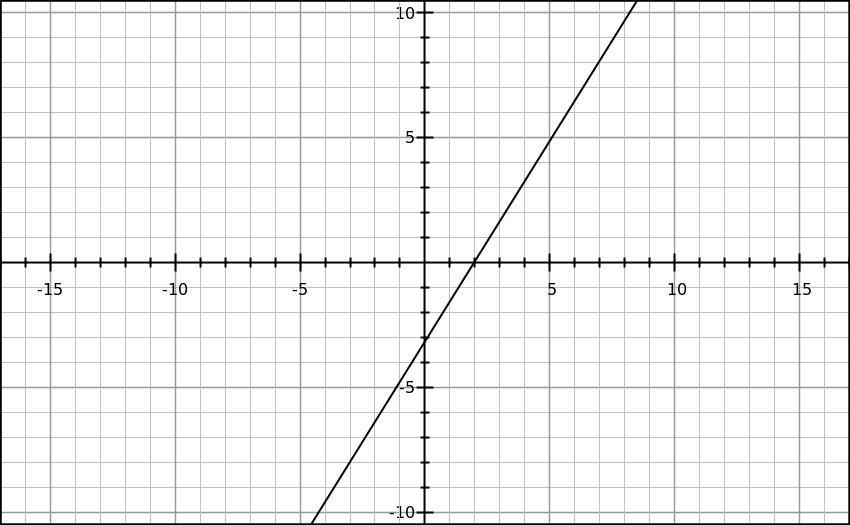Explanation:

First, solve for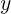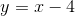.

Then, graph the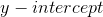at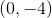.

Since the slope of the line is, you can graph the point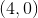as well.

There is only one graph that fits these requirements.### Example Question #1 : Cartesian Coordinate System

Which of the following does not lie on the line given by the equation below?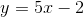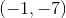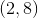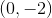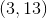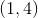Explanation:

To determine if a point lies on a line, plug in the x-value and y-value to see if the equation is satisfied. We can do this for each choice to check.

For example: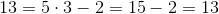Since both sides are equivalent, this point does lie on the line.

We can continue to do this for each of the points until one point does not work out.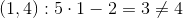Thus, this point does not lie on the line. Thus, this must be the solution.

### All Precalculus Resources